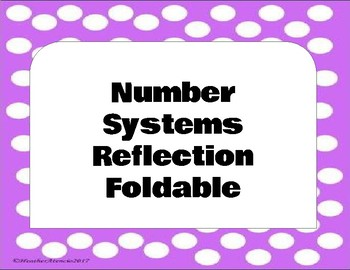# Number Systems Reflection Foldable 7Subject
Resource Type
File Type

PDF

(330 KB|2 pages)
Product Rating
Standards
• Product Description
• StandardsNEW
This page can be used after completing the 7th grade unit on Number Systems. Students can add this page in their math notebook as a unit divider. Each common core geometry standard is written in an "I Can" statement to help the student understand if they accomplished the standard. Students color in if they always, sometimes, or not yet met the standard. Sheet should be printed on color paper to stick out as a divider in the student's notebook.
Solve real-world and mathematical problems involving the four operations with rational numbers.
Convert a rational number to a decimal using long division; know that the decimal form of a rational number terminates in 0s or eventually repeats.
Apply properties of operations as strategies to multiply and divide rational numbers.
Understand that integers can be divided, provided that the divisor is not zero, and every quotient of integers (with non-zero divisor) is a rational number. If 𝘱 and 𝘲 are integers, then –(𝘱/𝘲) = (–𝘱)/𝘲 = 𝘱/(–𝘲). Interpret quotients of rational numbers by describing real-world contexts.
Understand that multiplication is extended from fractions to rational numbers by requiring that operations continue to satisfy the properties of operations, particularly the distributive property, leading to products such as (–1)(–1) = 1 and the rules for multiplying signed numbers. Interpret products of rational numbers by describing real-world contexts.
Total Pages
2 pages
N/A
Teaching Duration
N/A
Report this Resource to TpT
Reported resources will be reviewed by our team. Report this resource to let us know if this resource violates TpT’s content guidelines.